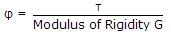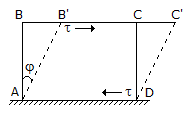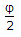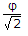# Civil Engineering - UPSC Civil Service Exam Questions

31.

A particle of mass 3 kg moving in a straight line decelerates uniformly from a speed of 40 m/s to 20 m/s in a distance of 300 m. Before it comes to rest, it will travel a further distance pf

 A. 10 m B. 20 m C. 50 m D. 100 m

Explanation:

No answer description available for this question. Let us discuss.

32.

For designing end bearing piles of square cross-section in clays having average unconfmed compressive strength of 6 t/m2, the net ultimate bearing capacity may be taken as

 A. 15 t/m2 B. 18 t/m2 C. 20 t/m2 D. 27 t/m2

Explanation:

No answer description available for this question. Let us discuss.

33.

The side AD of the square block ABCD as shown in the given figure is fixed at the base and it is under a state of simple shear causing shear stress τ and shear strain π, whereThe distorted shape is AB'C'D. The diagonal strain (linear) will beA.B.C. φ2 D. φ

Explanation:

No answer description available for this question. Let us discuss.

34.

Consider the following statements :
Collision diagram is used to
1. study accident pattern
2. eliminate accidents
3. determine remedial measures
4. make statistical analysis of accidents
Of these statements :

 A. 1 and 2 are correct B. 1 and 3 are correct C. 3 and 4 are correct D. 2 and 4 are correct

Explanation:

No answer description available for this question. Let us discuss.

35.

Consider the following statements :
1. An ascending gradient of 1 in 100 meets an ascending gradient of 1 in 120 to form a Valley curve.
2. A falling gradient of 1 in 25 meets a falling gradient of 1 in 50 to form a summit curve.
3. The length of summit curve is determined on the basis of head light sight distance.
Which one of the statements given above is/are correct ?

 A. 1 and 2 B. 2 and 3 C. 1 and 3 D. 2 only